GNSS Geodesy Innovation Interviews UAV/UAS Positioning Surveying Applications Perspective Time Navigation Mapping Policy Land Administration News Archives LBS GIS SDI Imaging E-ZineGeodesy

# Determination of local gravimetric geoid

Feb 2007 | Comments Off on Determination of local gravimetric geoid

###### A case study of Delhi area

The demand for a high resolution geoid model has grown substantially during the last few decades especially after inception of Global Positioning System (GPS). Many countries across the world have already developed their own geoidal model which serve as the means of deriving orthometric heights from GPS observations. The impact of GPS on surveying application is undeniable. More so, this revolution has not been confi ned to the surveying community, but has extended into mapping, navigation and Geographic information system (GIS) areas. During the last few years, we have been witnessing the wide spread adoption of GPS with an equivalently vibrant range of accuracy requirement. Many of these applications require accurate vertical positions.

The task of transforming the ellipsoidal height obtained from GPS technique to the orthometric height has prompted geodesists around the world to determine the high precision geoid undulations, for their region of interest. In India the present day nation wide geoid was computed a long time back and based on astro geodetic observations with respect to Everest spheroid. It has various limitations and does not have any signifi cance as far as GPS solutions for orthometric height is concerned.

Present study was taken up to validate the results of orthometrc heights derivation in a pilot project of large scale mapping of a part of Delhi through Airborne Laser Terrain Mapping(ALTM) Technique. A fairly dense gravity anomaly data consisting of about 160 uniformly distributed points covering a block of 1° X 1° including National Capital Region(NCR) of Delhi was used in the geoidal modelling process .The study was aimed at to analyse approach of data preparation and treatment procedures and a evaluation of test results obtained from the analytical solution of Stokes’ integral with appropriate Kernel modifi cations.

## Gravimetric Geoid and GPS

The geoid can be broadly defi ned an equipotential surface of Earth’s gravity fi eld that closely approximates with mean sea level (MSL) neglecting long term effect of sea surface topography (SST). The fundamental relationship between the geoid and
reference ellipsoid is given as:
h = H + N
Where h -> ellipsoidal height
H ->orthometric height Geoid
N-> separation between geoid ellipsoid
termed as geodial undulation. The relationship can be more clearly shown in fi gure 1.
Geoidal undulation (N) is required for many geodetic and surveying applications the most notable of these being the need for converting GPS-derived ellipsoidal height (h) to orthometric heights (H). The reference surface for orthometric heights was tradionally defi ned by the MSL measured at one or more tide gauges and realized through geodetic levelling in India for example the datum for orthmetric height was defi ned in 1909 using the MSL data furnished from 9 tide gauges sites at Karachi, Bombay, Karwar, Beypore, Cochin, Nagappattinam, Madras, Vishkhapatnam and false point (Burrard, S.G, 1910). The datum defi ned in 1909 is still in use and suffi ce most of the practical applications.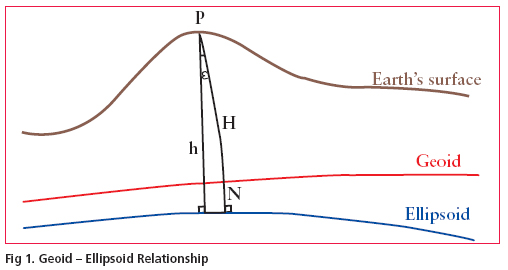The geoid undulations (N) may be computed in a simple manner by doing GPS observations in order to determine the ellipsoidal heights at all levelling bench marks. However if heights of some other points is required to be given it may not be possible to extrapolate the GPS- levelling heights differences. In this case gravimetric information may be used to bridge the gap through determination of local gravimetric geoid. In a broad sense geoidal surface is that undulating surface along which the potential remains the same.The undulations of the geoid are not the same as, but are affected by the variations in topography. Because of this complexity, high resolution gravimetric geoid models and associated interpolations software have been developed to support GPS height conversion.

–~~~~~~~~~~~~–

## Geoid computation procedure

The geoid determination process employed Stokes’ integral formulae which allows a pointwise calculation of gravity fi eld quantities and thus provide the possibility of an arbitrarily high gravity fi eld resolution which depends only on data coverage and quality. Utilizing local gravity anomalies as the primary data set classical solutions is aimed at the determination of geoid height.

Purely gravimetric calculations of geoid heights is hampered by longwave systematic data errors and by inhomogeneous spatial resolutions and accuracy of the local gravity data. The global geopotential models such as EGM96( Lemoine et.al.,1998) generally provides the long wave part of the gravity fi eld and dense local gravity data together with highresolutions digital elevation models leads to a combined solution that can be applicable to a limited region, where data smoothening techniques are used by considering the terrain effect. A remove compute- restore technique(Schwarz et.al.,1990) is applied in this study which includes the following steps.
1. Calculation of necessary corrections e.g. gravity formula corrections for free-air anomalies data to obtain corrected gravity anomalies Δgcor

2. Reduction of the gravity anomaliesΔgcor by the anomaly part of the global model to a degree of expansions m and obtain the reduced anomalies Δgm

3. Smoothening of the anomalies by applying the terrain corrections Δgt.

4. Griddings of the residual gravity anomalies
Δgres = Δg – Δgcor – Δgm – Δgt

5. Application of Stokes formula on the residual gravity anomalies resulting on residual geoid heights Nres

6. Restoration of the effect of the global model and the terrain to the residual geoid heights:
N= Nres +Nm +Nt
A schematic diagram of the general computation procedure is given in figure 2.

Stokes Integration:
The Stokes integral is one of the fundamental and most important formulae in physical geodesy. It was derived by G.G. Stokes in 1849 to compute geoid undulations N from terrestrial gravity anomalies (Heiskanen and Moritz, 1967) :(1)
γ Mean normal gravity for the earth
σ The sphere of integrations
S(ψ) Stokes` function

Δg Free air gravity anomalies dσ Element of surface area on the sphereΨ Surface spherical radius (ψ) between two point on the sphere and is given by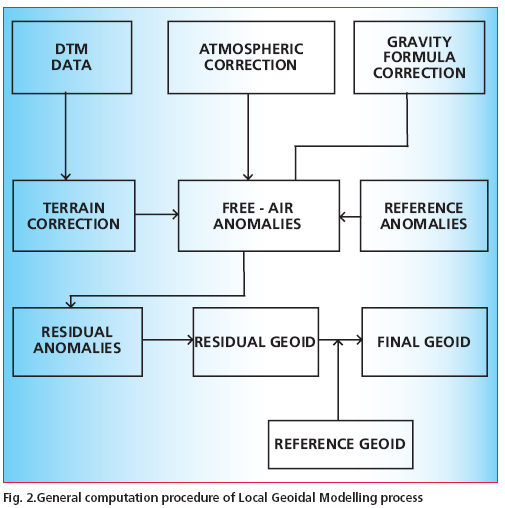cosψ = sinΦ sinΦ` + cosΦ
cosΦ` cos(λ` – λ)
Where Φ,λ are geographical coordinates of computation point and Φ`and λ` are the coordinates of surface element dσ. The Stokes` function in closed form is defi ned as,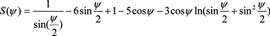(2)
The Stokes` formula in its original form suppresses the harmonic terms of degrees one and zero in N and it holds only for a reference ellipsoid that;
(1) Has the same potential as the geoid.
(2) Encloses a mass that is numerically equal to earth’s mass.
(3) Has its centre at the centre of gravity of the earth.

–~~~~~~~~~~~~–

## Data preparation

Free air gravity anomoly data
Free air anomaly values of test area were compiled from observed gravity database maintained by Geodetic & Research Branch (G&RB) , Survey of India. The compiled data base comprised of about 160 gravity values spread uniformly over the test area (see fi g.3). The datum for all Indian gravity measurement is a network of local base stations which were tied to the International gravity standardization Network 1971 (Morelli et. al. 1971). It is not known whether any variant of the tidal correction was applied to the local gravity observation and also the given elevations of gravity stations
were of variable quality. Some of the gravity observations were done on benchmarks of Indian levelling network whereas for a large portion of gravity stations the elevations were computed based on barometric levelling which can be in error of the order of ±2 – 4 m. Since the gravity anomalies values were based on International Gravity formula (1967) therefore to bring them in the Geodetic Reference System 1980 (GRS80) the following transformation was applied (NGS, 1986).
Δg1980 = Δg1967 – (0.8316 + 0.0782 sin2Ø – 0.0007 sin4Ø)

The converted values were used in subsequent computations of geoid model.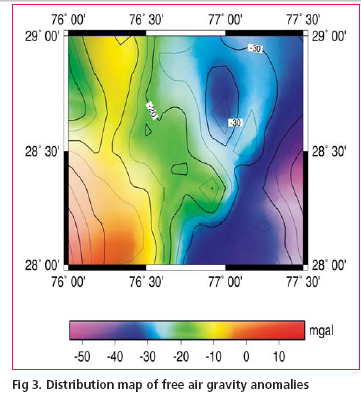Digitial elevation model for terrain correction
Since as on today no nationwide digitial elevation model (DEM) on appropriate scale is available, a local DEM was computed based on about 130 spot heights in the area. These elevation data have been gridded by using least square collocation (LSC) technique with second order Markov covariance function(Fig.4(a)). The DEM was generated in a grid of 15 X 15 arc second and tested for observed heights versus the interpolated heights. In general these spot heights were found to be in good agreement with the interpolated DEM heights.

## Methodology

The methodology for geoid computation was based on remove –compute– restore technique as described in sec.2. and can be summarized as follows:
• Remove the long wave length part of EGM96 global geopotential model and terrain effect from the observed free air gravity anomalies to derive residual gravity anomalies(Fig.5).
• Compute the residual undulations NRES of the geoid by numerical integration of Stokes integral (Eqn.1) using reduced gravity anomaly data.
• Restore the long wavelength effect NEGM96 and residual terrain effect NRTM to get final geoidal undulation N that is:
N = NEGM96 + NRTM + NRES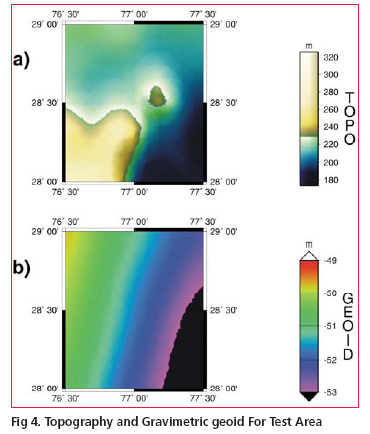Computation of geoidal height at a given point with respect to EGM96 (NEGM96) is quite simple using the EGM96 spherical harmonic potential Coeffi cient set and spherical harmonic correction coeffi cient both complete to degree and order 360. However the procedure for computation of indirect effect from local topography(NRTM)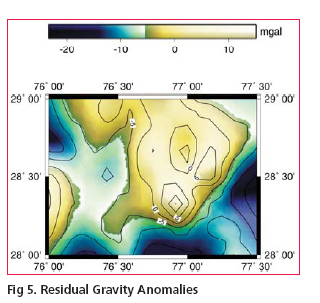–~~~~~~~~~~~~–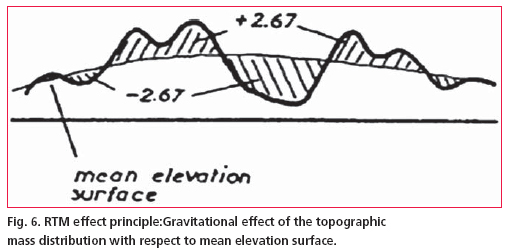and Residual undulation NRES using Stokes` integral is little complicated and required to be discussed in detail.

Terrain correction
Computation of NRTM is done relative to the mean elevation surface (fig.6) In case of remove – compute – restore technique, substracting the contribution of reference global geopotential model from the local terrestrial gravity data also include the effects of the global topography ; therefore substraction of further topographic effect may introduce long wave length effects into the residual potential. To avoid this only short wavelengths of the topographic effect may be used, which is termed as residual terrain model (RTM) effect(Forseberg,R,1994) . The RTM terrain effect may be computed in a spherical cap around the computation point, provided the cap is sufficiently large so that the remote residual topography has a negligible effect. In this study the mean elevation surface was determined from the DEM data by applying the moving average method. The RTM gravity terrain effect in the planner approximation given by a volume integral: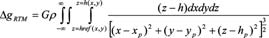(3)
Where h are the heights of topography, G is the gravitational constant andρ is the mass density taken as 2.67 gm/cm3 in the computation. The computations of ΔgRTM were done
in space domain prism integration by Fast Fourier Transform (FFT) method using the dense height data.

## Modifi cation of kernel

Modifi cation of Kernel S (ψ) in Stokes` formula forms an important part of geoid determination process due to the fact that long wave-length systematic errors in gravity data can produce large geoid errors. These systematic errors can be avoided by modifying the classical Stokes` Kernel in an appropriate manner. There are different ways of modifi cations, however in our study we used the modifi cation suggested by Wong and Gore (1996).

As per the technique the spheroidal Stokes’ kernel S(ψ) in equation (1), which is implicit to the Stokes’ formula, can be modifi ed simply by removing the appropriate-degree Legendre polynomials [Pn(cosψ)] from the closed form of the spherical Stokes’ Kernel (Eqn.2).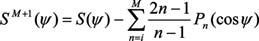(4)
Where is the spherical distance between the computation point and integration points. We may choose any degree of modifi cation to our choice which permits the ultimate geoid to best fi t the GPS-Levelling undulations. At the same time the
modifi cation approach should be applied in combination with capsize radius ψ = ψm as both the reference geoid EGM96 as well as local gravity data may have errors and therefore the difference between the two geoids i.e. gravimetric and GPS-Levelling with different degrees of modifi cations does not necessarily equal to zero.

Thus kernel modifi cation and capsize assumptions provide the means of optimising the solution of Stokes` integral in determination of local geoid.

## Geoid model construction

For computation of geoid the degree of Kernel modifi cation in Eqn. (4) was chosen to be m=360, which is same as the degree of reference global geopotential model EGM96. Eqn. (1) when applied over a limited spherical cap of radius (ψ°=0.5°) about each computation
point leads to the following approximation of geoid height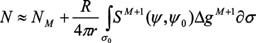(5)
The concept of spherical cap of limited spatial extent in analytical solutions of Stokes’ integration was implemented simply by setting the value of to zero out side the cap region. The fi nal geoid was constructed based on the methodology described in section 3. The reduced gravity data was arranged in a grid using Least Squares collocation (LSC) technique. Geoidal heights were computed by applying the generalized Stokes’ scheme(Eqn. 5) using spherical cap of radius 0.5°. NRTM was computed by planar approximation implemented using FFT technique on the 0.5 Km basic resolution grid. Finally, NEGM96 was added to NRES and NRTM to obtain the final geoid (Fig. 4(b)). Table 1 below shows the statistics of the various component computed at the different stages of the model construction Looking at the table-1 the effect of Residual terrain model (NRTM) is almost negligible and only shortwave residual gravity anomalies contributes to reference geoid i.e. EGM96. However the effect, as evident from the table-1 is order of 35-55 cm which is a minor quantity in comparison to the total geoidal undulation (≈- 53 m). Thus EGM96 geoid is very smooth in the region and almost fit in to the local geoid having only the minor short wave length variations.

–~~~~~~~~~~~~–

## Evaluation of Geoid Model

Differences between the GPS derived ellipsoidal heights and the levelling heights of bench mark of national control network are generally being used for geoid evaluation. In present study the GPS/levelling differences were derived by making GPS observations on 50 nos. of stations and connecting them to bench marks of Indian vertical control network by running precision leveling lines. GPS data was processed using BERNESE Software Ver.4.2 and an accuracy of derived ellipsoidal height of the order of few cm has been achieved. None of these stations was included in the geoid modeling process. The quantities to compare are the geoidal heights from the gravimetric geoid Ngrav and the corresponding geoidal height NGPS from GPS/leveling observations. The misfi t ε ε = NGPS – Ngrav includes datum differences, systematic errors and subsidence /uplift in the levelling, as well as errors in the gravimetric geoid. The statistics of the GPS/levelling differences and heights from gravimetric geoidal model have been presented in Table 2 and Fig. 7 shows the contour plot of the differences. The above results clearly show a close matching between the gravimetric geoid and GPS/leveling differences and probably described the advantage of using dense gravity data. There is hardly any longitudinal variations noticed for the geoid but it has shown the traces of northward gradual slope which may be evident more clearly when computations are taken for a larger region. Though the results still contain the systematic differences between local levelling network and the gravimetric geoid but major contribution of these errors has been nullified due to the fact that test area is considerably small and terrain effect is almost negligible because of smooth topography of the region.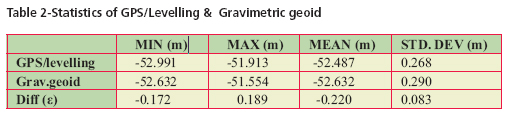## Conclusions

This paper has described a brief review of some of the important aspects involved in computation of gravimetric geoid model in Indian context. The use of generalized scheme along with modifi ed Stokes Kernel in remove-compute-restore technique has been successful to a great extent in computation of geoid model from dense gravity anomaly data of Delhi area. The study has fi rmly shown that EGM96 can be effectively used for gravimetric geoidal modeling in India notwithstanding its own shortcomings provided that the other aspects of the methodology are explicitly designed and followed carefully. The procedure of analytical solution of Stokes integrations with spherical cap of radius of o.5° using dense gravity data has worked well and achieved an accuracy, in absolute sense, of the order of 20 cm as determined from comparison with GPS/leveling differences making it an alternative to conventional method of leveling, suitable for most of the mapping applications. However a signifi cant size of errors in GPS determined ellipsoidal heights is always expected from the various error sources. Even with a very detailed error modeling of all the possible source effects the achievable accuracy of GPS ellipsoidal height is always considered to be less than the horizontal positional accuracy. Hence the extent of misfit between the gravimetric geoid and GPS/levelling difference should not be always viewed entirely due to error in gravity measurements or inadequate geoidal modelling procedure.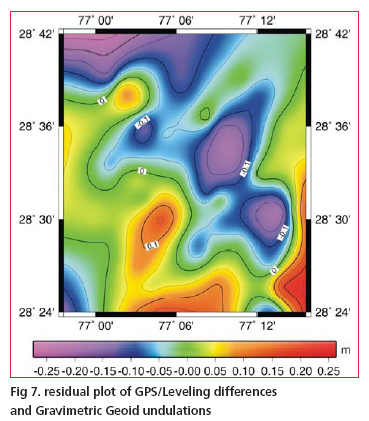## Acknowledgements

The authors gratefully acknowledge the consistent effort made by officers and staff of satellite geodesy and project survey wings of G&RB for their excellent work of field data collection, processing and typing of this manuscript.

## References

Burrared, S.G.,1910, Levelling of Precision in India, GTS Vol. XIX, Survey of India, Dehradun

Forsberg ,R.(1994) Terrain effects in Geoid computation, Lecture notes, Int. school for the determination and use of geoid, Milano.

Heiskanen, W,Moritz , H (1967) Physical Geodesy, Freeman, San Fransisco.

Lemoine FG, Smith D, Smith R, Kunz L, Pavlis E, Pavlis N, Klosko S, Chinn D, Torrence M,Williomson R, Cox C, Rachlin K, Wang Y, Kenyon S, Salman R, Trimmer R, Rapp R, Nerem S (1996) The development of NASA GSFC and DMA joint Geopotential model, Proc. of International Symposium on Gravity Geoid and Marine Geodesy, University of Tokyo.

Morelli C,Gantar C,Honcaslo T,Mc connel RK,Tanner TG,Szabo B,Uotila,U,Whalen CT(1971) The International Gravity Standardisation Network(IGSN71),Bull
Geod.Sp. publ 4.

Schwarz K.P., Sideris M.G. and Forsberg R. (1990).The uses of FFT techniques in Physical Geodesy. Geophysical J. Int, pp.485-514.

Vanicek P , Sjöberg LE(1991) Reformulation of Stokes’ theory for higher than second-degree reference fi eld and modifi cation of integration kernels,J. Geophys. Res. 96(B4):6529-6540.## S K Singh

###### gandrb@vsnl.net.in## Brig (Dr) B Nagarajan

###### Branch, Survey of India,## P K Garg

##### INDUSTRY | LBS | GPS | GIS  | REMOTE SENSING | GALILEO UPDATE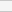(No Ratings Yet)Loading...